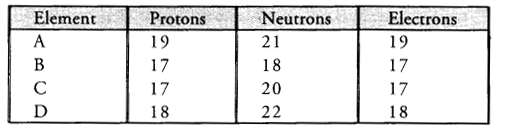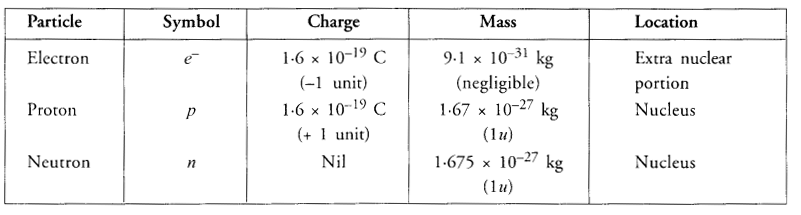# HOTS Questions for Class 9 Science Chapter 4 Structure of the Atom

## HOTS Questions for Class 9 Science Chapter 4 Structure of the Atom

These Solutions are part of HOTS Questions for Class 9 Science. Here we have given HOTS Questions for Class 9 Science Chapter 4 Structure of the Atom

Question 1.
Both helium (He) and beryllium (Be) have two valence electrons. Whereas He represents a noble gas element, Be does not. Assign reason.
The element He (Z = 2) has two electrons present in the only shell i.e., K-shell. Since this shell can have a maximum of two electrons only therefore, He is a noble gas element. The element Be (Z = 4) has electronic configuration as : 2, 2. Although the second shell has also two electrons but it is not complete. It can still accommodate six more electrons. Therefore, the element beryllium does not represent a noble gas element.

More Resources

Question 2.
Study the data given below and answer the questions which follow :1. Write the mass number and atomic number of the particles A, B, C and D.
2. Which represent a pair of isotopes ?

1. Particle A : Mass number = 7 ; Atomic number = 3
Particle B : Mass number = 17 ; Atomic number = 9
Particle C : Mass number =16, Atomic number = 8
Particle D : Mass number =18, Atomic number = 8
2. Particles C and D represent a pair of isotopes since they have same atomic number.

Question 3.
Which of the two will be chemically more reactive ; element X with atomic number 17 or element Y with atomic number 16 ?
The electronic configuration of the two elements are as follows :
X(Z = 16): K (2), L(8), M(6) ;
Y(Z = 17): K(2), L(8), M(7)
The element X needs two electrons in the M shell to have the noble gas configuration of element, Ar (Z = 18). However, the element Y needs only one electron to achieve this. This means that the element Y has a greater urge or desire to take up one electron from an outside atom. It is therefore, more reactive than the element X which needs two electrons.

Question 4.
The number of protons, neutrons and electrons in particles from A to E are given below :1. Which one is a cation ?
2. Which one is an anion ?
3. Which represent pair of isotopes ?

1. B is a monovalent cation (B+)
2. E is a monovalent anion (E)
3. A and D represent pair of isotopes.

Question 5.
An atom of an element has three electrons in the third shell which is the outermost shell. Write

1. the electronic configuration
2. the atomic number
3. number of protons
4. valency
5. the name of the element
6. its nature whether metal or non-metal. (CBSE 2012)